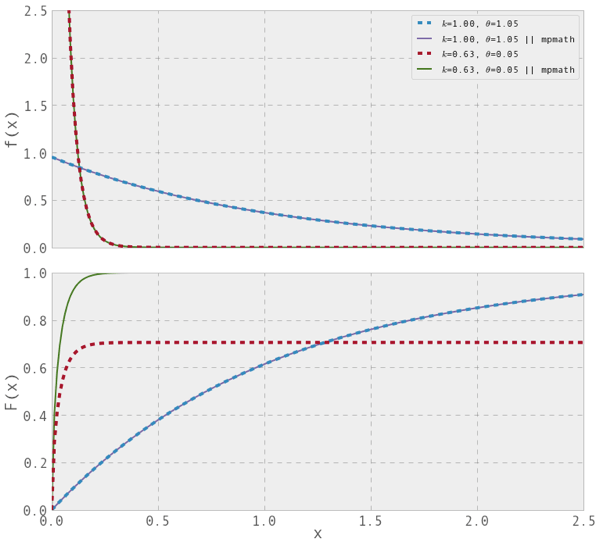# planetwater

ground- water, geo- statistics, environmental- engineering, earth- science

## Gamma Function in Scipy.Special

I was playing with the Gamma Distribution Function in python. As typically, I used a combination of bumpy numpy arrays and the scipy.special functions for Gamma and incomplete gamma functions. At one point I realized that the Gamma distribution function (bottom panel on the figure below) was not reaching unity for large x values and for some parameters (k=0.63, theta=0.05). In the given case, the deviation was about 0.3 in probability space, which is significant.

Note: The Gamma Distribution Function (its cdf or pdf) is not the same thing as the Gamma function!

The solution I came up with is to use the module mpmath. When I do, everything looks fine.

I googled only briefly, but did not find any description of this error in scipy.special. Also I am not sure, if using mpmath is the best/fastest/most elegant solution. However, at least it seems to work.Written by Claus

August 19th, 2013 at 3:05 pm

Posted in

### 4 Responses to 'Gamma Function in Scipy.Special'

1. I get the same strange plots if I define the cdf of the gamma distribution as:

gamma_cdf = lambda x, alpha, beta: sp.special.gammainc(alpha, beta * x) / sp.special.gamma(alpha)

or, if you like the other formulation better:

gamma2 = lambda x, k, beta: gamma_cdf(x, k, 1 / beta)

which is the obvious thing to do. However in scipy.special, gammainc is defined as:

“1 / gamma(a) * integral(exp(-t) * t**(a-1), t=0..x)”

So, dividing by sp.special.gamma(alpha) is the straw that broke the gamma’s back. This will bring you up to 1:

gamma_cdf = lambda x, alpha, beta: sp.special.gammainc(alpha, beta * x)

By the way: are “bumpy arrays” more uneven than numpy ones? SCNR (I am also sorry for the url)gamma gamma gamma gamma gamma gammeleon

19 Aug 13 at 5:51 pm

2. well, gamma gamma, thanks for pointing out what breaks ones neck.. 😉Claus

19 Aug 13 at 7:00 pm

3. Things may get even hairier when you use more complex distributions in scipy.stats.

One can get quite interesting insights into some distributions – after the headaches of transforming parameters from textbooks or wikipedia into loc, scale, etc. of the stats functions to achieve the same results, have gone away.

I think scipy.stats.gamma is one of those candidates.

By the way, why don’t you use that one?Dommy

10 Sep 13 at 11:14 am

4. thanks for pointing towards the functions integrated into scipy.stats — I guess I wanted to see things more directly… 😉Claus

14 Sep 13 at 3:54 pm